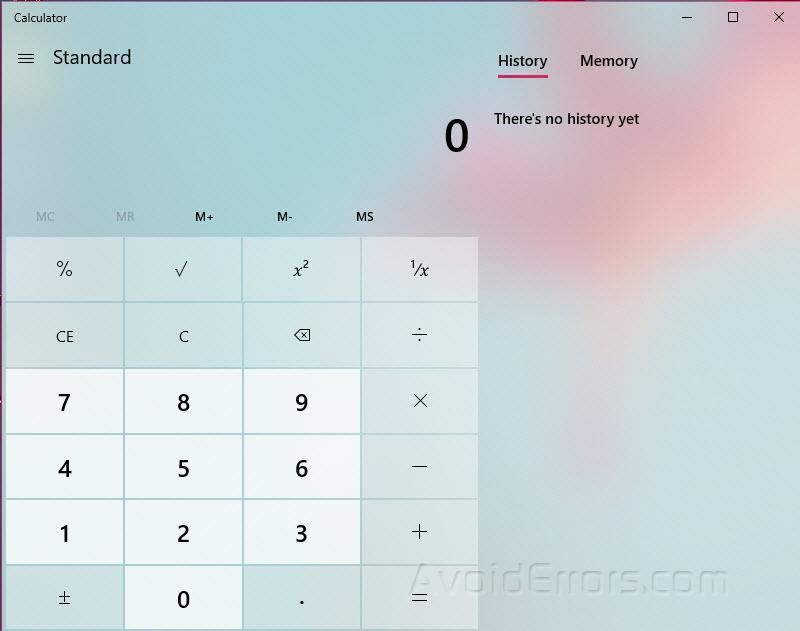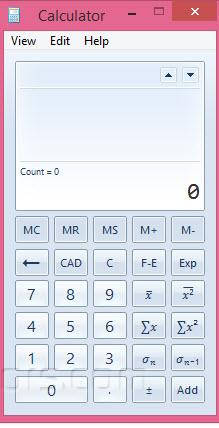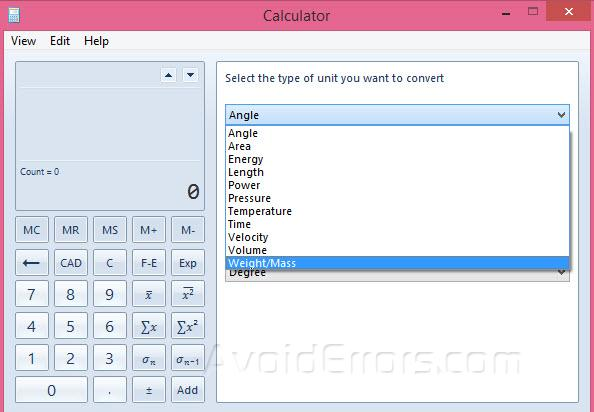# How to Use Advance Mode Calculator – Windows 10

The Calculator app for Windows 10 is a friendly version of the desktop calculator which works on both mobile and desktop devices. You can open multiple instances of calculators at the same time on the desktop and can switch between the Standard, Scientific, Programmer, Date calculation, and Converter modes of the app.

To start, select the Start button, and then select Calculator from the list of apps.### Standard Mode:

The Standard mode, gives the ability to perform basic math functions like addition, subtraction, multiplication, and division.

There is the option to calculate square root, store numbers in the memory, and percentages. This mode is great for figuring out day-to-day math problems or tallying numbers.### Scientific Mode:

The Scientific mode gives you the functions of a standard scientific calculator. To switch to scientific mode click on View > Scientific Calculator or press Alt + 2.

In this mode you will find functions like Sin, Cos, Tan, Degrees, Radians, Grads etc. to perform the scientific calculations that you want.### Programmer mode:

The programmer calculator is for computer science persons. To get to this mode, click on View > Programmer Calculator or press Alt + 3. In this mode, you can switch between Hexadecimal, Decimal, Octal, and Binary number systems. You’ll see things like RoR, And, Rsh which are functions like logic gates and bit shifting.### Statistical mode:

The statistics calculator will be useful for statistical analysis. You find the average function, an average of the square of values, the sum of the values, sum of the square of the values, standard deviation, and standard deviation of the population. All these tools are basic to statistical mathematics.### Math with Dates:

For planning events or anything date-related, you can use date calculation function. You can calculate the difference between two dates, add or subtract days from a specific date etc.### Conversion of Measurement Units:

The unit conversion mode can compute angle, area, energy, length, power, pressure, temperature, time, velocity, volume, and weight and mass conversions. It can convert several different units of measurement within each category.